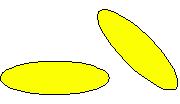Email us to get an instant 20% discount on highly effective K-12 Math & English kwizNET Programs!

#### Online Quiz (WorksheetABCD)

Questions Per Quiz = 2 4 6 8 10

### Grade 4 - Mathematics12.14 Geometry Part Three - Review Test

 Q 1: In the figure shown are the two figures similar?YesNo Q 2: What is the area of a rectangle with length 27 ft and width 24 ft648 sq cm648 sq ft648 sq inches Q 3: Are these figures congruent?YesNo Q 4: What is the perimeter of a rectangle with length 8 cm and width 7 cm?50 cm56 cm15 cm30 cm Q 5: How many lines of symmetry does a circle have?101590more than you can count Q 6: A rectangular prism has 2 layers of 8 cubes. What is the volume?32 cubic units16 cubic units10 cubic units Q 7: What is the area of a rectangle with length 5 inches and width 9 inches14 sq inches4 sq inches45 sq inches Q 8: Which is not a solidrectangletriangular pyramidrectangular prismcylinder Question 9: This question is available to subscribers only! Question 10: This question is available to subscribers only!